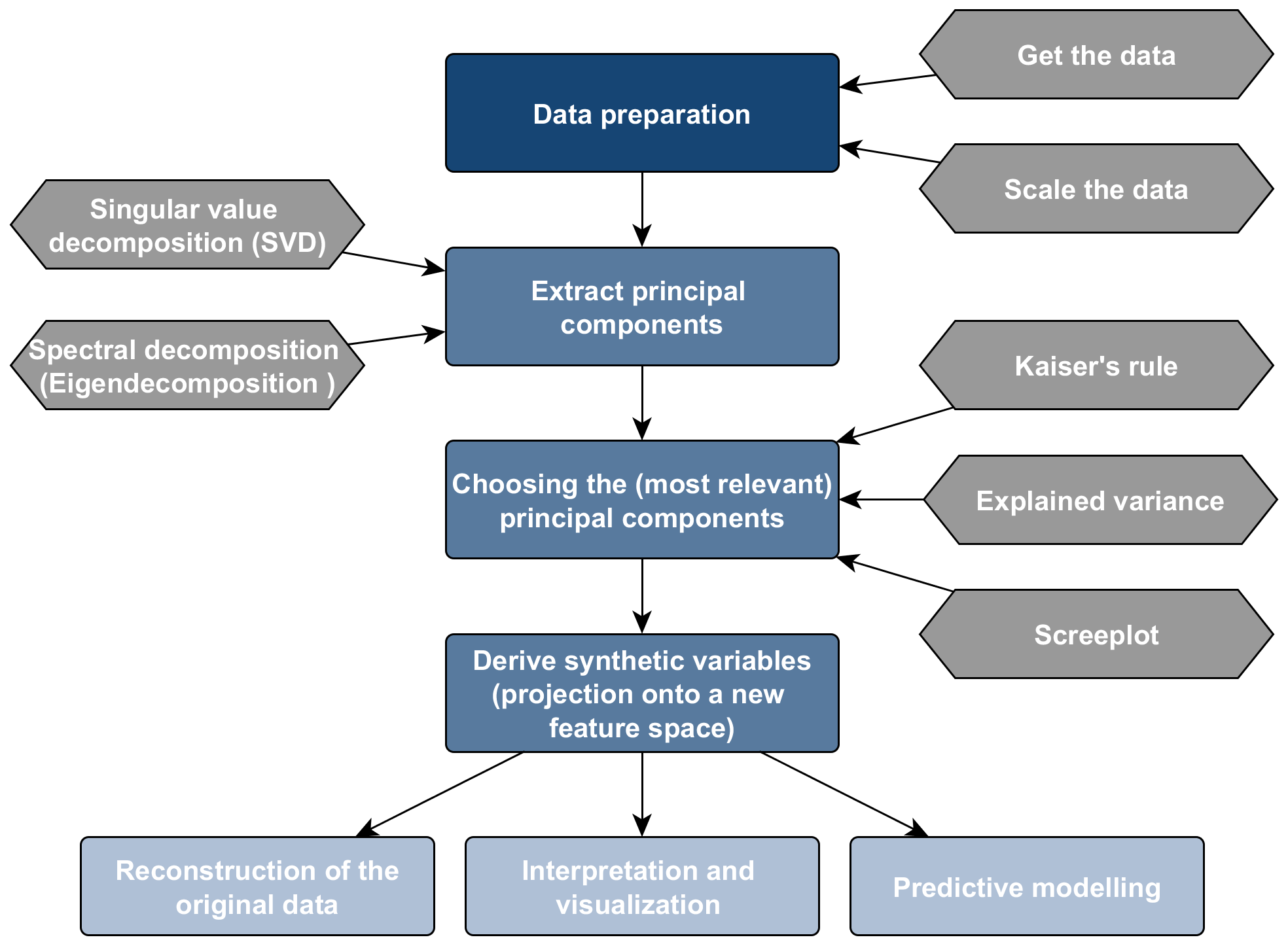Principal component analysis (PCA) allows us to summarize a set of features with a smaller number of representative features that collectively explain most of the variability in the original data set. PCA projects the observations described by $$d$$ features into orthogonal, and thus by definition uncorrelated, variables. The new set of synthetic variables is equal in number to the original set. However, the first synthetic variable represents as much of the common variation of the original variables as possible, the second variable represents as much of the residual variation as possible, and so forth.

PCA is particularly powerful in dealing with multicollinearity and variables that outnumber the samples $$(d > n )$$, and it is widely used for explanatory data analysis, outlier detection and as a data pre-processing technique for predictive modelling. The figure below outlines the analysis workflow.PCA Workflow